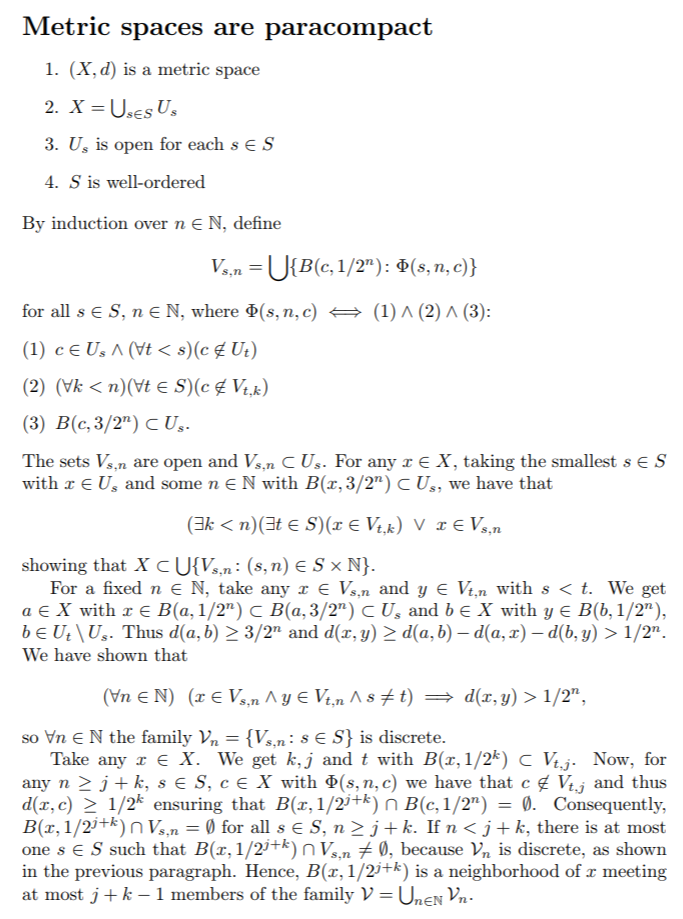# Proof that metric spaces are paracompact

• metric-spaces-are-paracompact.pdf
• metric-spaces-are-paracompact.tex\documentclass[10pt]{article} \usepackage{amssymb,amsmath,amsthm} \usepackage{enumerate} \newcommand*{\N}{\ensuremath{\mathbb{N}}} \begin{document} \section*{Metric spaces are paracompact} \begin{enumerate} \item $(X,d)$ is a metric space \item $X = \bigcup_{s\in S}U_s$ \item $U_s$ is open for each $s\in S$ \item $S$ is well-ordered \end{enumerate} \noindent By induction over $n\in\N$, define $V_{s,n}=\bigcup\{B(c,1/2^n)\colon \Phi(s,n,c)\}$ for all $s\in S$, $n\in\N$, where $\Phi(s,n,c)\iff(1)\wedge(2)\wedge(3)$: \begin{enumerate}[(1)] \item $c\in U_s\wedge(\forall t<s)(c\not\in U_t)$ \item $(\forall k<n)(\forall t\in S)(c\not\in V_{t,k})$ \item $B(c,3/2^n)\subset U_s$. \end{enumerate} The sets $V_{s,n}$ are open and $V_{s,n}\subset U_s$. For any $x\in X$, taking the smallest $s\in S$ with $x\in U_s$ and some $n\in\N$ with $B(x,3/2^n)\subset U_s$, we have that $(\exists k<n)(\exists t\in S)(x\in V_{t,k})\ \vee\ x\in V_{s,n}$ showing that $X\subset\bigcup\{V_{s,n}\colon (s,n)\in S\times\N\}$. For a fixed $n\in\N$, take any $x\in V_{s,n}$ and $y\in V_{t,n}$ with $s<t$. We get $a\in X$ with $x\in B(a,1/2^n)\subset B(a,3/2^n)\subset U_s$ and $b\in X$ with $y\in B(b,1/2^n)$, $b\in U_t\setminus U_s$. Thus $d(a,b)\geq 3/2^n$ and $d(x,y)\geq d(a,b)-d(a,x)-d(b,y)>1/2^n$. We have shown that $(\forall n\in\N)\ \ (x\in V_{s,n}\wedge y\in V_{t,n}\wedge s\not=t)\implies d(x,y)>1/2^n,$ so $\forall n\in\N$ the family $\mathcal{V}_n=\{V_{s,n}\colon s\in S\}$ is discrete. Take any $x\in X$. We get $k,j$ and $t$ with $B(x,1/2^k)\subset V_{t,j}$. Now, for any $n\geq j+k$, $s\in S$, $c\in X$ with $\Phi(s,n,c)$ we have that $c\not\in V_{t,j}$ and thus $d(x,c)\geq 1/2^k$ ensuring that $B(x,1/2^{j+k})\cap B(c,1/2^n)=\emptyset$. Consequently, $B(x,1/2^{j+k})\cap V_{s,n}=\emptyset$ for all $s\in S$, $n\geq j+k$. If $n<j+k$, there is at most one $s\in S$ such that $B(x,1/2^{j+k})\cap V_{s,n}\not=\emptyset$, because $\mathcal{V}_n$ is discrete, as shown in the previous paragraph. Hence, $B(x,1/2^{j+k})$ is a neighborhood of $x$ meeting at most $j+k-1$ members of the family $\mathcal{V}=\bigcup_{n\in\N}\mathcal{V}_n$. \end{document}